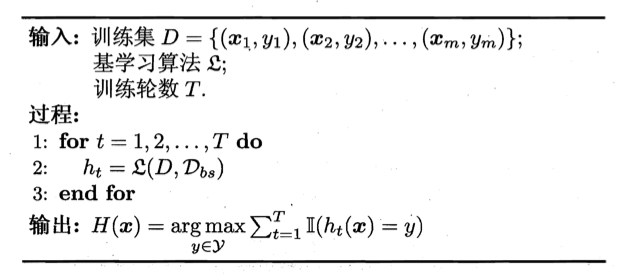# 【机器学习基础】第二十九课：集成学习之Bagging与随机森林

## Bagging，“包外估计”（out-of-bag estimate），随机森林（Random Forest）

Posted by x-jeff on November 9, 2021

【机器学习基础】系列博客为参考周志华老师的《机器学习》一书，自己所做的读书笔记。

# 1.前言

【机器学习基础】第二十七课：集成学习之个体与集成可知，欲得到泛化性能强的集成，集成中的个体学习器应尽可能相互独立；虽然“独立”在现实任务中无法做到，但可以设法使基学习器尽可能具有较大的差异。给定一个训练数据集，一种可能的做法是对训练样本进行采样，产生出若干个不同的子集，再从每个数据子集中训练出一个基学习器。这样，由于训练数据不同，我们获得的基学习器可望具有比较大的差异。然而，为获得好的集成，我们同时还希望个体学习器不能太差。如果采样出的每个子集都完全不同，则每个基学习器只用到了一小部分训练数据，甚至不足以进行有效学习，这显然无法确保产生出比较好的基学习器。为解决这个问题，我们可考虑使用相互有交叠的采样子集。

# 2.Bagging$\mathcal{D}_{bs}$是自助采样产生的样本分布。

Bagging是一个很高效的集成学习算法。值得一提的是，自助采样过程还给Bagging带来了另一个优点：由于每个基学习器只使用了初始训练集中约63.2%的样本，剩下约36.8%的样本可用作验证集来对泛化性能进行“包外估计”（out-of-bag estimate）。为此需记录每个基学习器所使用的训练样本。不妨令$D_t$表示$h_t$实际使用的训练样本集，令$H^{oob}(\mathbf x)$表示对样本$\mathbf x$的包外预测，即仅考虑那些未使用$\mathbf x$训练的基学习器在$\mathbf x$上的预测，有：

$H^{oob}(\mathbf x)=\arg \max \limits_{y \in \mathcal{Y}} \sum^T_{t=1} \mathbb{I} (h_t(\mathbf x)=y) \cdot \mathbb{I} (\mathbf x \notin D_t)$

$\epsilon^{oob} = \frac{1}{\lvert D \rvert} \sum_{(\mathbf x,y)\in D} \mathbb{I} (H^{oob}(\mathbf x) \neq y)$

# 3.随机森林